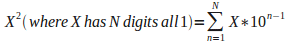Toward a Unified Metaphysical Understanding: Interesting countup-countdown pattern
 Interesting countup-countdown pattern
 2011-02-25, by John Ringland Taking numbers consisting of 1's and squaring them reveals an interesting countup-countdown pattern in the resulting product. 1^2= 1 11^2= 121 111^2= 12321 1111^2= 1234321 11111^2= 123454321111111^2= 12345654321 1111111^2= 1234567654321 11111111^2= 123456787654321 111111111^2= 12345678987654321 1111111111^2= 1234567900987654321 11111111111^2= 123456790(12)0987654321 111111111111^2= 123456790(1232)0987654321 1111111111111^2= 123456790(123432)0987654321 11111111111111^2= 123456790(12345432)0987654321 111111111111111^2= 123456790(1234565432)0987654321 1111111111111111^2= 123456790(123456765432)0987654321 11111111111111111^2= 123456790(12345678765432)0987654321 111111111111111111^2= 123456790(1234567898765432)0987654321 1111111111111111111^2= 123456790(123456790098765432)0987654321 11111111111111111111^2= 123456790(123456790(12)098765432)0987654321 111111111111111111111^2= 123456790(123456790(1232)098765432)0987654321 1111111111111111111111^2= 123456790(123456790(123432)098765432)0987654321 11111111111111111111111^2= 123456790(123456790(12345432)098765432)0987654321 ... The pattern continues indefinitely with countup-countdown 'bubbles' emerging in the middle each time that the previous countup-countdown pattern reaches completion. Numbers have such intriguing patterns! Lets find out why this pattern occurs, in terms of the algebra involved... For 2 digits: 11 * 11 = 11 + 11 + 11 + 11 + 11 + 11 + 11 + 11 + 11 + 11 + 11 = (11 + 11 + 11 + 11 + 11 + 11 + 11 + 11 + 11 + 11) + 11 = (10 * 11) + 11 = ```110 + 11 --- 121``` Because 11 = 10 + 1 we could split of one 11 and group together the other ten 11's. Furthermore, because we happen to be using base 10 numerals, this splitting off the (10*11) is equivalent to shifting them all across by one digit. Then when the shifted and unshifted values are added they cross over in such a way that they create ascending then descending values in the final result. For 3 digits: 111 * 111 = 111 + 111 + ... (total of 111 times) = (100 * 111) + (10 * 111) + 111 = ```11100 + 1110 111 ----- 12321``` Notice how we could break up the sum into groups of 100, 10 and 1, which correspond to different shift values. Notice how the rows shift across each other and add down the columns to give ascending then descending values in the result. In generalTo quickly test this, if N = 3 then 111^2 = 111 + 1110 + 11100 = 12321 So the pattern occurs because the fact that 11 = 10 + 1 and the fact that we are using base 10 numerals both combine to create a shifting addition effect that results in ascending and descending values in the final result. Thanks to Maria Celeste G for bringing this general pattern to my attention and thanks to Michael Schirner for hinting at why the pattern exists. On the subject on numerical patterns, here is another one, Some Intriguing Mathematical Properties of the Mayan Calendar

 [< Back] [Toward a Unified Metaphysical Understanding]

Category:   Tags:

Other entries tagged as ""
2013-12-08: Motivating and Clarifying the Paradigm Shift at the Heart of Science
2013-12-02: What is the highest perceived benefit or aspiration of my Life?
2013-11-28: The world-view arising from my work
2013-11-26: Motivation behind my work
2013-11-26: Quantum Mechanics, Naïve Realism, Scientific Realism, Abstraction and Reality
2013-11-24: Reformulation of the Virtual Reality Hypothesis
2012-05-09: Regarding the nature of reality and the 'world'
2012-05-08: Questions regarding information and process
2012-05-02: Computational Paradigm 101
2012-05-01: Summary of the main 'products' of my research

Other entries in
2013-12-08: Motivating and Clarifying the Paradigm Shift at the Heart of Science
2013-12-02: What is the highest perceived benefit or aspiration of my Life?
2013-11-30: Cognitive Repression in Physics - Reasons for the Entrenched Culture of Denial
2013-11-28: The world-view arising from my work
2013-11-26: Motivation behind my work
2013-11-26: Quantum Mechanics, Naïve Realism, Scientific Realism, Abstraction and Reality
2013-11-24: Reformulation of the Virtual Reality Hypothesis
2013-11-11: What is a differend?
2013-10-29: Freedom, Slavery and Fundamental Limits on the Growth of Civilisation
2012-05-31: What is the relationship between processes and systems?

 [< Back] [Toward a Unified Metaphysical Understanding] [PermaLink]?